# Next Sentence Prediction using BERT

• Last Updated : 27 Jan, 2022

Pre-requisite: BERT-GFG

BERT stands for Bidirectional Representation for Transformers. It was proposed by researchers at Google Research in 2018. Although, the main aim of that was to improve the understanding of the meaning of queries related to Google Search. A study shows that Google encountered 15% of new queries every day. Therefore, it requires the Google search engine to have a much better understanding of the language in order to comprehend the search query.

However, BERT is trained on a variety of different tasks to improve the language understanding of the model. In this article, we will discuss the tasks under the next sentence prediction for BERT.

### Next Sentence Prediction Using BERT

BERT is fine-tuned on 3 methods for the next sentence prediction task:

• In the first type, we have sentences as input and there is only one class label output, such as for the following task:
• MNLI (Multi-Genre Natural Language Inference): It is a large-scale classification task. In this task, we have given a pair of sentences. The goal is to identify whether the second sentence is entailment, contradiction, or neutral with respect to the first sentence.
• QQP (Quora Question Pairs): In this dataset, the goal is to determine whether two questions are semantically equal.
• QNLI (Question Natural Language Inference): In this task, the model needs to determine whether the second sentence is the answer to the question asked in the first sentence.
• SWAG (Situations With Adversarial Generations): This dataset contains 113k sentence classifications. The task is to determine whether the second sentence is the continuation of the first or not.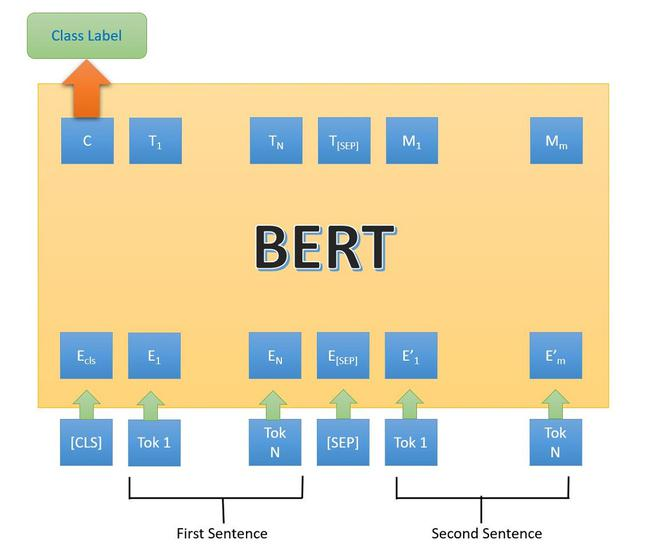BERT architecture first type

• In the second type, we have only one sentence as input, but the output is similar to the next class label. Following are the task/datasets used for it:
• SST-2 (The Stanford Sentiment Treebank): It is a binary sentence classification task consisting of sentences extracted from movie reviews with annotations of their sentiment representing in the sentence. BERT generated state-of-the-art results on SST-2.
• CoLA: (Corpus of Linguistic Acceptability): is the binary classification task. The goal of this task to predict whether an English sentence that is provided is linguistically acceptable or not.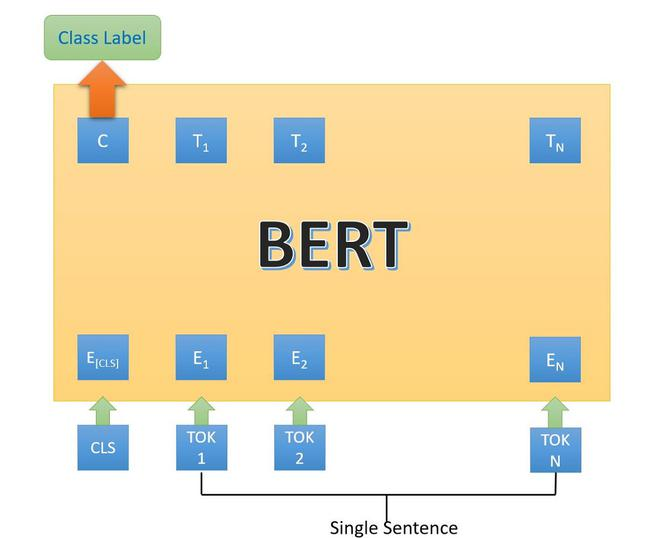BERT architecture second type

• In the third type of next sentence, prediction, we have been provided with a question and paragraph and outputs a sentence from the paragraph that is the answer to that question. It is performed on SQuAD (Stanford Question Answer D) v1.1 and 2.0 datasets.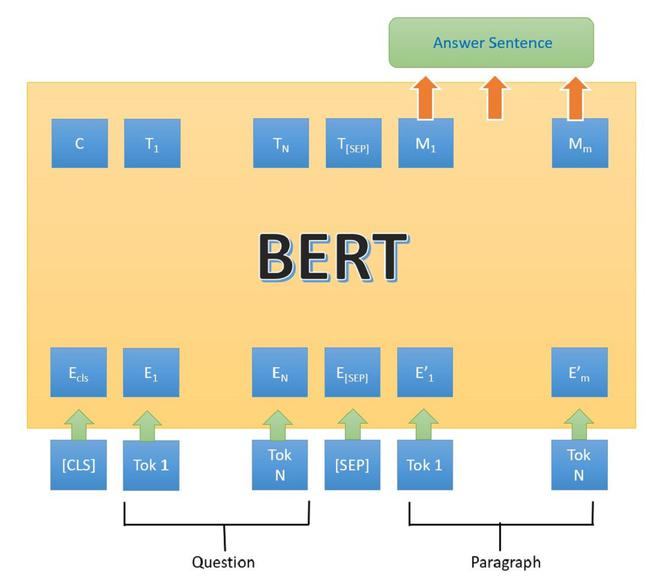BERT architecture 3rd type.

In the above architecture, the [CLS] token is the first token in the input. This means an input sentence is coming, the [SEP] represents the separation between the different inputs. Here, the inputs sentence are tokenized according to BERT vocab, and output is also tokenized.

### Implementation

• In this implementation, we will be using the Quora Insincere question dataset in which we have some question which may contain profanity, foul-language hatred, etc. We will be using BERT from TF-dev.

## Python3

 `# Check if there is GPU or not``!nvidia``-``smi``# Install tensorflow 2.3.0``!pip install ``-``q tensorflow``=``=``2.3``.``0``# Clone the TensorFlow models Repo``!git clone ``-``-``depth ``1` `-``b v2.``3.0` `https:``/``/``github.com``/``tensorflow``/``models.git``!pip install ``-``Uqr models``/``official``/``requirements.txt``# Imports``import` `sys``import` `numpy as np``import` `tensorflow as tf``import` `tensorflow_hub as hub``sys.path.append(``'models'``)``from` `official.nlp.data ``import` `classifier_data_lib``from` `official.nlp.bert ``import` `tokenization``from` `official.nlp ``import` `optimization` `# keras imports``from` `tf.keras.layers ``import` `Input``, Dropout, Dense``from` `tf.keras.optimizers ``import` `Adam``from` `tf.keras.metrics ``import` `BinaryAccuracy``from` `tf.keras.losses ``import` `BinaryCrossentropy``from` `tf.keras.utils ``import` `plot_model``from` `tf.keras.models ``import` `Model``# Load the Quora Insincrere QUesrtion dataset.``df ``=` `pd.read_csv(``  ``'https://archive.org/download/fine-tune-bert-tensorflow-train.csv/train.csv.zip'``,``                 ``compression``=``'zip'``)``df.head()``# plot the histogram of sincere and insincere question vs sincere ques``df.target.plot(kind``=``'hist'``, title``=``'Sincere (0) vs Insincere (1) distribution'``)`

```qid question_text target
000002165364db923c7e6 How did Quebec nationalists see their province...0
1000032939017120e6e44 Do you have an adopted dog, how would you enco...0
20000412ca6e4628ce2cf Why does velocity affect time? Does velocity a...0
3000042bf85aa498cd78e How did Otto von Guericke used the Magdeburg h...0
40000455dfa3e01eae3af Can I convert montra helicon D to a mountain b...0```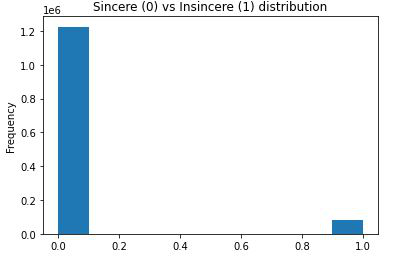Sincere vs Insincere

• In the code below, we will be using only 1% of data to fine-tune our Bert model (about 13,000 examples), we will be also converting the data into the format required by BERT and to use eager execution, we use a python wrapper. Before doing this, we need to tokenize the dataset using the vocabulary of BERT.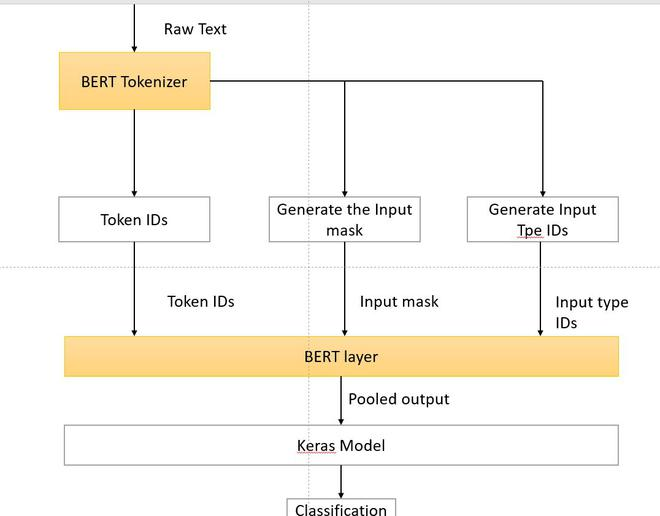## Python3

 `# split into train and validation``train_df, remaining ``=` `train_test_split(df, train_size``=``0.01``,``                                       ``stratify``=``df.target.values)``valid_df, _ ``=` `train_test_split(remaining,  train_size``=``0.001``,``                               ``stratify``=``remaining.target.values)``train_df.shape, valid_df.shape` `# import for processing dataset``from` `tf.data.Dataset ``import` `from_tensor_slices``from` `tf.data.experimental ``import` `AUTOTUNE` `# convert dataset into tensor slices``with tf.device(``'/cpu:0'``):``  ``train_data ``=``from_tensor_slices((train_df.question_text.values,``                                                   ``train_df.target.values))``  ``valid_data ``=` `from_tensor_slices((valid_df.question_text.values,``                                                   ``valid_df.target.values))``    ` `  ``for` `text, label ``in` `train_data.take(``2``):``    ``print``(text)``    ``print``(label)``    ` `label_list ``=` `[``0``, ``1``] ``# Label categories``max_seq_length ``=` `128` `# maximum length of input sequences``train_batch_size ``=` `32` `# Get BERT layer and tokenizer:``bert_layer ``=` `hub.KerasLayer(``  ``"https://tfhub.dev/tensorflow/bert_en_uncased_L-12_H-768_A-12/2"``,``                            ``trainable``=``True``)``vocab_file ``=` `bert_layer.resolved_object.vocab_file.asset_path.numpy()``do_lower_case ``=` `bert_layer.resolved_object.do_lower_case.numpy()``tokenizer ``=` `tokenization.FullTokenizer(vocab_file, do_lower_case)` `# example``# convert to tokens ids and``tokenizer.convert_tokens_to_ids(``  ``tokenizer.wordpiece_tokenizer.tokenize(``'how are you?'``))` `# convert the dataset into the format required by BERT i.e we convert the row into``# input features (Token id, input mask, input type id ) and labels` `def` `convert_to_bert_feature(text, label, label_list``=``label_list,``               ``max_seq_length``=``max_seq_length, tokenizer``=``tokenizer):``  ``example ``=` `classifier_data_lib.InputExample(guid ``=` `None``,``                                            ``text_a ``=` `text.numpy(),``                                            ``text_b ``=` `None``,``                                            ``label ``=` `label.numpy())``  ``feature ``=` `classifier_data_lib.convert_single_example(``0``, example, label_list,``                                    ``max_seq_length, tokenizer)``  ` `  ``return` `(feature.input_ids, feature.input_mask, feature.segment_ids,``          ``feature.label_id)` `# wrap the dataset around the python function in order to use the tf``# datasets map function``def` `to_bert_feature_map(text, label):``  ` `  ``input_ids, input_mask, segment_ids, label_id ``=` `tf.py_function(``    ``convert_to_bert_feature,``    ``inp``=``[text, label],``    ``Tout``=``[tf.int32, tf.int32, tf.int32, tf.int32])` `  ``# py_func doesn't set the shape of the returned tensors.``  ``input_ids.set_shape([max_seq_length])``  ``input_mask.set_shape([max_seq_length])``  ``segment_ids.set_shape([max_seq_length])``  ``label_id.set_shape([])` `  ``x ``=` `{``        ``'input_word_ids'``: input_ids,``        ``'input_mask'``: input_mask,``        ``'input_type_ids'``: segment_ids``    ``}``  ``return` `(x, label_id)``with tf.device(``'/cpu:0'``):``  ``# train``  ``train_data ``=` `(train_data.``map``(to_bert_feature_map,``                              ``num_parallel_calls``=``AUTOTUNE)``                          ``#.cache()``                          ``.shuffle(``1000``)``                          ``.batch(``32``, drop_remainder``=``True``)``                          ``.prefetch(AUTOTUNE))` `  ``# valid``  ``valid_data ``=` `(valid_data.``map``(to_bert_feature_map,``                            ``num_parallel_calls``=``AUTOTUNE)``                          ``.batch(``32``, drop_remainder``=``True``)``                          ``.prefetch(AUTOTUNE))` `# example format train and valid data``print``(``"train data format"``,train_data.element_spec)``print``(``"validation data format"``,valid_data.element_spec)`

```((13061, 3), (1293, 3))

#printed an example
tf.Tensor(b'What is your experience living in Venezuela in the current crisis? (2018)', shape=(), dtype=string)
tf.Tensor(0, shape=(), dtype=int64)

# converted to tokens
['how', 'are', 'you', '?']
[2129, 2024, 2017, 29632]

# train and validation data
# train
({'input_mask': TensorSpec(shape=(32, 128), dtype=tf.int32, name=None),
'input_type_ids': TensorSpec(shape=(32, 128), dtype=tf.int32, name=None),
'input_word_ids': TensorSpec(shape=(32, 128), dtype=tf.int32, name=None)},
TensorSpec(shape=(32,), dtype=tf.int32, name=None))

# validation
({'input_mask': TensorSpec(shape=(32, 128), dtype=tf.int32, name=None),
'input_type_ids': TensorSpec(shape=(32, 128), dtype=tf.int32, name=None),
'input_word_ids': TensorSpec(shape=(32, 128), dtype=tf.int32, name=None)},
TensorSpec(shape=(32,), dtype=tf.int32, name=None))```
• In this step, we will wrap the BERT layer around the Keras model and fine-tune it for 4 epochs, and plot the accuracy.

## Python3

 `# define the keras model``# Building the model``def` `fine_tuned_model():``  ``input_word_ids ``=` `Input``(shape``=``(max_seq_length,), dtype``=``tf.int32,``                                      ``name``=``"input_word_ids"``)``  ``input_mask ``=` `Input``(shape``=``(max_seq_length,), dtype``=``tf.int32,``                                  ``name``=``"input_mask"``)``  ``input_type_ids ``=` `Input``(shape``=``(max_seq_length,), dtype``=``tf.int32,``                                  ``name``=``"input_type_ids"``)` `  ``pooled_output, sequence_output ``=` `bert_layer([input_word_ids, input_mask,``                                               ``input_type_ids])` `  ``drop ``=` `Dropout(``0.4``)(pooled_output)``  ``output ``=` `Dense(``1``, activation``=``"sigmoid"``, name``=``"output"``)(drop)` `  ``model ``=` `Model(``    ``inputs``=``{``        ``'input_word_ids'``: input_word_ids,``        ``'input_mask'``: input_mask,``        ``'input_type_ids'``: input_type_ids``    ``},``    ``outputs``=``output)``  ``return` `model` `#compile the model``model ``=` `fine_tuned_model()``model.``compile``(optimizer``=``Adam(learning_rate``=``2e``-``5``),``              ``loss``=``BinaryCrossentropy(),``              ``metrics``=``[BinaryAccuracy()])``model.summary()``#plot the model``plot_model(model``=``model, show_shapes``=``True``)``# Train model``epochs ``=` `4``history ``=` `model.fit(train_data,``                    ``validation_data``=``valid_data,``                    ``epochs``=``epochs,``                    ``verbose``=``1``)``# plot the accuracy``def` `plot_graphs(history, metric):``  ``plt.plot(history.history[metric])``  ``plt.plot(history.history[``'val_'``+``metric], '')``  ``plt.xlabel(``"Epochs"``)``  ``plt.ylabel(metric)``  ``plt.legend([metric, ``'val_'``+``metric])``  ``plt.show()``plot_graphs(history, ``'binary_accuracy'``)`

```Model: "functional_1"
__________________________________________________________________________________________________
Layer (type)                    Output Shape         Param #     Connected to
==================================================================================================
input_word_ids (InputLayer)     [(None, 128)]        0
__________________________________________________________________________________________________
input_mask (InputLayer)         [(None, 128)]        0
__________________________________________________________________________________________________
input_type_ids (InputLayer)     [(None, 128)]        0
__________________________________________________________________________________________________
keras_layer (KerasLayer)        [(None, 768), (None, 109482241   input_word_ids
input_type_ids
__________________________________________________________________________________________________
dropout (Dropout)               (None, 768)          0           keras_layer
__________________________________________________________________________________________________
output (Dense)                  (None, 1)            769         dropout
==================================================================================================
Total params: 109,483,010
Trainable params: 109,483,009
Non-trainable params: 1
__________________________________________________________________________________________________```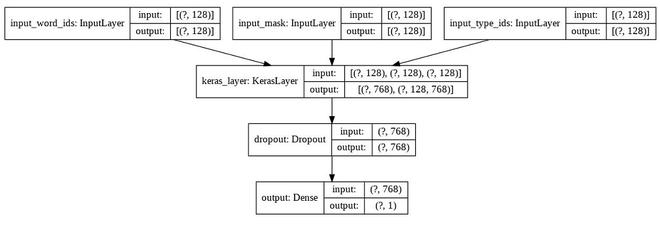Keras model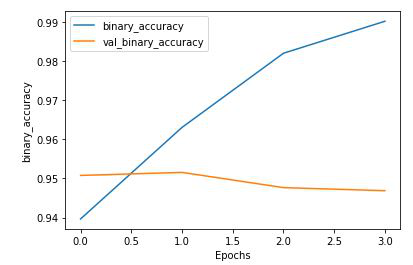Plot of Binary Accuracy

## Python3

 `# check``test_eg``=``[``'what is the current marketprice of petroleum?'``,``         ``'who is Oswald?'``, ``'why are you here idiot ?'``]``test_data ``=``from_tensor_slices((test_eg, [``0``]``*``len``(test_eg)))``# wrap test data into BERT format``test_data ``=` `(test_data.``map``(to_feature_map_bert).batch(``1``))``preds ``=` `model.predict(test_data)``print``(preds)``[``'Insincere'` `if` `pred >``=``0.5` `else` `'Sincere'` `for` `pred ``in` `preds]`

```[[1.3862031e-05]
[6.7259348e-04]
[8.9223766e-01]]
['Sincere', 'Sincere', 'Insincere']```

### Reference:

My Personal Notes arrow_drop_up